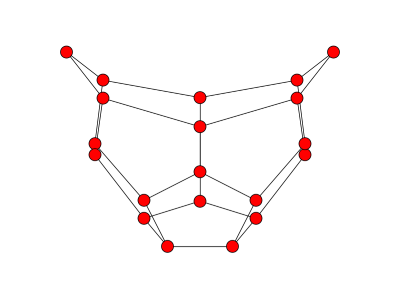# Graph regularity and Laplace eigenvalues

You can tell whether a graph is regular, or nearly regular, by looking at its eigenvalues.

Let G be a graph with n vertices and m edges and let L be the Laplacian matrix of G. Then the sum of the eigenvalues of L is 2m. (The diagonal of L contains the degrees of each node, so it’s trace is twice the number of edges. And the trace of a matrix is the sum of its eigenvalues.)

Let λi be the eigenvalues of the L. Then we havewith equality if and only if G is regular. [reference]

We can try this out by starting with a dodecahedron graph. It’s a regular graph, since each vertex has three edges. There are a total of 30 edges and the eigenvalues sum to 60. The right-most expression is 60 as expected.Next remove one of the edges from the graph. The result bears a remarkable resemblance to Iron Man.Now there are 29 edges, so 2m = 58. The right-most expression above is now 58.31. It’s bigger than 58 because the new graph is not regular. But as expected, it’s close to 58 since the graph is close to regular.